### Eric M. Schauber, Brett J. Goodwin, Clive G. Jones, and Richard S. Ostfeld. 2007. Spatial selection and inheritance: applying evolutionary concepts to population dynamics in heterogeneous space. Ecology 88:1112–1118.

Appendix A. Derivation of spatial inheritability, spatial selective differential, and change in per capita population growth rate.

Here, we derive expressions for spatial inheritability (IS2) for both two-patch and continuous-space models and derive Eq. 4 from the population growth equations of both models.

Two-patch model

The definition of IS2 (Eq. 1) yields:(A.1)

where wi is the suitability of patch i. It can further be shown that: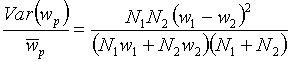(A.2)

where Ni is the number of individuals inhabiting patch i. The population model projecting generation t+1 (offspring) from generation t (parent) for patches i = 1,2 and ji is defined by:

 Ni,t+1 = (1 – b)wiNi,t + bwjNj,t (A.3)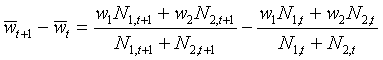(A.4)

Substituting Eq. A.3 into Eq. A.4, applying Eqs. A.1 and A.2, and rearranging yields: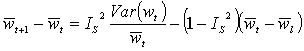(A.6)

which is identical to Eq. 4.

Continuous-space model

In deriving for the continuous-space model, we again consider a regression analysis in the case of no selection (parents randomly distributed in space, all parents produce equal numbers of offspring, and offspring disperse randomly). The expected covariance between offspring and parental fitness depends on the autocorrelation of environmental fitness between generations as a function of distance:(A.7)

where k(x) is the dispersal kernel (probability density function of an offspring dispersing distance x), wo and wp are fitnesses of an individual offspring and its parent respectively, wx is suitability at a location distance x away from the parental location, and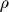(x) is the autocorrelation of suitability between generations at lag distance x. This framework is based on assumptions that offspring dispersal distance follows an invariant dispersal kernel (no response to local population density or fitness) and that the autocorrelation of suitability depends on distance only (not on direction or location in space). As with the two-patch model:(A.8)

Expected fitness of offspring (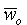) is governed by the mean fitness of parental generation ( ), the relative offspring production of parents at each location, and the heritability of location-associated fitness. It is the weighted average of the expected offspring fitness of parents over all locations, where weighting is the relative offspring production. Thus, the change in mean fitness is given by:(A.9)

Applying Eqs. A.7 and A.8 to Eq. A.9 and rearranging yields: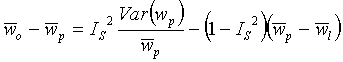(A.10)

which is identical to Eq. 4.

[Back to E088-069]